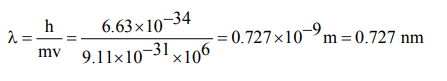## Atomic Structure Questions and Answers Part-8

1. The value of the energy for the first excited state of hydrogen atom will be
a) –13.6 eV
b) –3.40 eV
c) –1.51 eV
d) –0.85 eV

Explanation:2. The radius of hydrogen atom in the ground state is 0.53Å. The radius of $Li^{2+}$ ion (atomic number = 3) in a similar state is
a) 0.17 Å
b) 1.06 Å
c) 0.53 Å
d) 0.265 Å

Explanation:3. The Bohr orbit radius for the H-atom (Z=1) is approximately 0.53 Å. The radius for the first excited state orbit is
a) 0.13 Å
b) 1.06Å
c) 4.77 Å
d) 2.12 Å

Explanation:4. The ratio of the radius of the first Bohr orbit for the electron orbiting the hydrogen nucleus to that of the electron orbiting the deuterium nucleus (mass nearly twice that of the hydrogen nucleus) is approximately
a) 2 : 1
b) 1 : 1
c) 1 : 2
d) 4 : 1

Explanation:5. The energy of the first electron in helium will be
a) –13.6 eV
b) –54.4 eV
c) –5.44 eV
d) zero

Explanation:6. Who modified Bohr’s theory by introducing eiliptical orbits for electron path?
a) Hund
b) Thomson
c) Rutherford
d) Sommerfeld.

Explanation: Sommerfield

7. Wavelength associated with electron motion
a) increases with increase in speed of electron
b) remains same irrespective of speed of electron
c) decreases with increase of speed of $\overline{e}$ (electron)
d) is zero

Explanation:8. If the Planck’s constant $h=6.6×10^{-34}Js$    , the de Broglie wavelength of a particle having momentum of 3.3 × 10–24 kg ms–1 will be
a) 0.002 Å
b) 0.5Å
c) 2Å
d) 500Å

Explanation:9. What is the wavelength associated with an electron moving with a velocity of $10^{6}m/s$    ? given $\left( h=6.63 ×10^{-34} Js , m=9.11 × 10^{-31}Kg\right)$
a) 72.7 nm
b) 0.727 nm
c) 7.27 nm
d) None of thesea) $10^{-10}m$
b) $10^{-20}m$
c) $10^{-30}m$
d) $10^{-40}m$